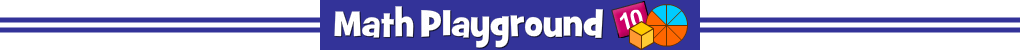AdvertisementPenguin Jump Multiplication
NUMBER OF PLAYERS: 4
The penguins are glacier hopping again. How fast can they go?
Practice multiplication facts and power up your penguin pal.
Penguin Jump Multiplication - Learning Connections
Essential Skills
Mental Math - multiply numbers by memory or through other efficient methods
Problem Solving - match multiplication expressions with the correct products

Common Core Connection for 3rd Grade
Determine the unknown whole number in a multiplication equation (4 x 6 = ?).
Apply properties of operations as strategies to multiply.
Know from memory all products of two one-digit numbers.
More Math Games to Play